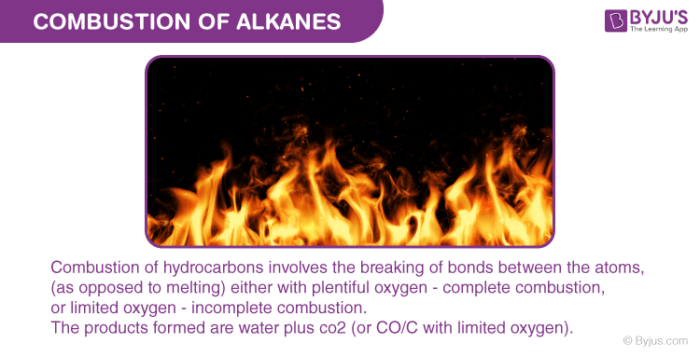Checkout JEE MAINS 2022 Question Paper Analysis : Checkout JEE MAINS 2022 Question Paper Analysis :

# Combustion Of Hydrocarbons : AlkanesLet us first understand the terms combustion and alkanes individually then move towards the topic. Combustion or burning is a high temperature exothermic reaction. It happens between a fuel and oxygen (oxidant), giving out gaseous products, also termed as smoke. Alkane is referred to as a saturated open chain hydrocarbon consisting of carbon-carbon single bonds.

### Combustion of alkanes:

1. Complete combustion of alkanes: When alkane is heated in the presence of sufficient air or dioxygen it forms carbon dioxide and water and enormous amount of heat energy is released. For example:

CH4 (g)   +   2O2 (g) → CO2 (g) + 2H2O (l);                                ∆cHΘ = – 890 kJ mol-1

C4H10        + 13/2 O2(g) → 4CO2 (g) + 5H2O (l);                         ∆cHΘ = – 2875.84 kJ mol-1

The above reaction can be generalized as follows:

CnH2n+2   +   ((3n+1)/2) O2 nCO2   +   (n+1) H2O

Alkanes can also be used as a fuel, as they produce large amounts of heat.

1. Incomplete combustion of alkanes: It occurs when there is not enough amount of oxygen for fuel to react completely. This leads to the formation of carbon or carbon monoxide. Carbon monoxide formed as a by product is a colourless poisonous gas. For example:

$$\begin{array}{l}~~~~~~~~~~~~~~~\end{array}$$
Methane + (little) Oxygen –> Carbon + Water

$$\begin{array}{l}~~~~~~~~~~~~~~~\end{array}$$
CH4 + O2 → C + 2H2O

$$\begin{array}{l}~~~~~~~~~~~~~~~\end{array}$$
Methane + (some) Oxygen →  Carbon Monoxide + Water

$$\begin{array}{l}~~~~~~~~~~~~~~~\end{array}$$
CCH4(g)   +   (3/2)O2 (g)  →   CO(g)  + 2H2O(l)

The carbon black produced during the incomplete burning of alkane is used in the manufacturing of inks.

### Trends in combustion:

For a hydrocarbon, if complete ignition occurs then it burns with a blue flame. As the molecular mass of hydrocarbon increases it starts burning with a yellow coloured flame showing incomplete burning. The burning of hydrocarbons becomes difficult with increasing molecular weights.

Know more about Pyrolysis of Alkanes

Test your Knowledge on Combustion of hydrocarbons alkanes!